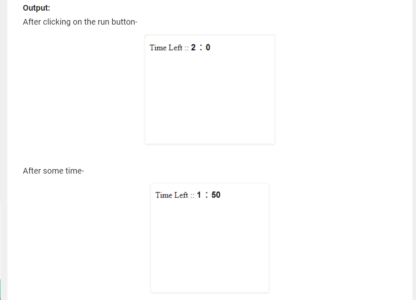# All you Need to Know about Timers in JavaScriptTimers are an important aspect of any programming language. As we know time is money. So in this article, we will see how to implement and work with Timers in JavaScript:

## Working with Timers in JavaScript

A timer is a function that enables us to execute a function at a particular time. Using timers you can delay the execution of code so that it does not get done at the exact moment an event is triggered or the page is loaded. For example, you can use timers to change the advertisement banners on your website at regular intervals, or display a real-time clock, etc.

There is a timer function in JavaScript: `setTimeout()`

The following section will show you how to create timers to delay code execution as well as how to perform one or more actions repeatedly using theis function in JavaScript.

## Window setTimeout() Method

Definition and Usage:

The setTimeout() method calls a function or evaluates an expression after a specified number of milliseconds.

TIP:

• 1000 ms = 1 second.
• The function is only executed once. If you need to repeat execution, use the `setInterval()` method.
• Use the `clearTimeout()` method to prevent the function from running.

This function accepts two parameters: a function, which is the function to execute, and an optional delay parameter, which is the number of milliseconds representing the amount of time to wait before executing the function (1 second = 1000 milliseconds).

Return Value: It returns a number representing the ID value of the timer that is set.

## Code: Timers in JavaScript

Here is the code for Timers in JavaScript that sets the timer of 2 minutes and when the times up the Page alert “times up”. The `setTimeout()` method calls a function or evaluates an expression after a specified number of milliseconds.

`<html> `

`<head> `

`<script> `

`//set minutes `

`var mins = 2; `

`//calculate the seconds `

`var secs = mins * 60; `

`//countdown function is evoked when page is loaded `

`function countdown() { `

`setTimeout('Decrement()', 60); `

`} `

`//Decrement function decrement the value. `

`function Decrement() { `

`if (document.getElementById) { `

`minutes = document.getElementById("minutes"); `

`seconds = document.getElementById("seconds"); `

`//if less than a minute remaining `

`//Display only seconds value. `

`if (seconds < 59) { `

`seconds.value = secs; `

`} `

`//Display both minutes and seconds `

`//getminutes and getseconds is used to `

`//get minutes and seconds `

`else { `

`minutes.value = getminutes(); `

`seconds.value = getseconds(); `

`} `

`//when less than a minute remaining `

`//colour of the minutes and seconds `

`//changes to red `

`if (mins < 1) { `

`minutes.style.color = "red"; `

`seconds.style.color = "red"; `

`} `

`//if seconds becomes zero, `

`//then page alert time up `

`if (mins < 0) { `

`alert('time up'); `

`minutes.value = 0; `

`seconds.value = 0; `

`} `

`//if seconds > 0 then seconds is decremented `

`else { `

`secs--; `

`setTimeout('Decrement()', 1000); `

`} `

`} `

`} `

`function getminutes() { `

`//minutes is seconds divided by 60, rounded down `

`mins = Math.floor(secs / 60); `

`return mins; `

`} `

`function getseconds() { `

`//take minutes remaining (as seconds) away `

`//from total seconds remaining `

`return secs - Math.round(mins * 60); `

`} `

`</script> `

`</head> `

`<!-- onload function is evoke when page is load --> `

`<!--countdown function is called when page is loaded --> `

`<body onload="countdown();"> `

`<div> `

`Time Left :: `

`<input id="minutes" type="text" style="width: 10px; `

`border: none; font-size: 16px; `

`font-weight: bold; color: black;"><font size="5"> : `

`</font> `

`<input id="seconds" type="text" style="width: 20px; `

`border: none; font-size: 16px; `

`font-weight: bold; color: black;"> `

`</div> `

`</body> `

`</html> `When less than a minute is remaining, the timer color changes to red.With this, we come to an end of this Timers in JavaScript article. Check out the Angular Certification Training by Edureka, a trusted online learning company with a network of more than 250,000 satisfied learners spread across the globe.

Got a question for us? Please mention it in the comments section of this Dependency Injection in AngularJs and we will get back to you.REGISTER FOR FREE WEBINAR
Send OTPThank you for registering Join Edureka Meetup community for 100+ Free Webinars each month

### Subscribe to our Newsletter, and get personalized recommendations.#### All you Need to Know about Timers in JavaScript

edureka.co